• 2021-05-31 03:33:25

目的: 发出 y = x ** 3 / (x * 3 + 1) 的图像

1. 导入matplotlib模块还有numpy模块:

import numpy as np
import matplotlib.pyplot as plt

2. 设置一个x的大致范围, 和y相对应的值域:

x = np.arange(-100, 100, 0.001)
y = x ** 3 / (3 * x + 1)


3. 使用figure创建画布, 把参数传进plot里面:

plt.figure()
plt.plot(x, y, linestyle='--', color='red')

4. 因为这个函数的范围太大, 并且在三分之一处出现断崖分布, 必须得缩小x轴和y轴的范围, 不然画图的误差会相当大:

lt.xlim((-0.6, 0.6))
plt.ylim((-0.6, 0.6))

5. 对图像进行些许的美化:

ax = plt.gca()
ax.spines['right'].set_color('none')
ax.spines['top'].set_color('none')
ax.xaxis.set_ticks_position('bottom')
ax.yaxis.set_ticks_position('left')
ax.spines['bottom'].set_position(('data', 0))
ax.spines['left'].set_position(('data', 0))

6. 最后要使用show展示出来:

plt.show()

7. 效果如下: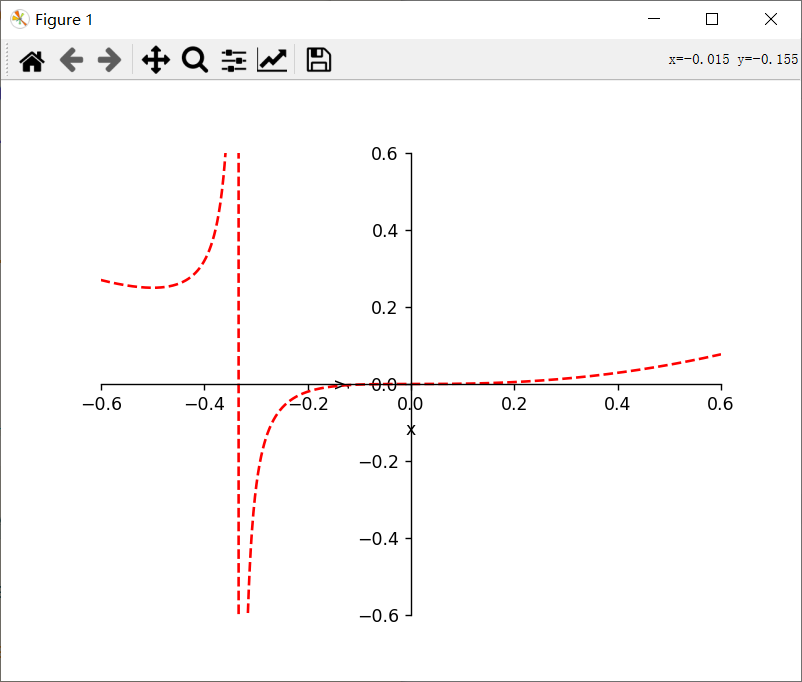8. 所用代码:

import numpy as np
import matplotlib.pyplot as plt

x = np.arange(-100, 100, 0.001)
y = x ** 3 / (3 * x + 1)

plt.figure()
plt.plot(x, y, linestyle='--', color='red')
plt.xlim((-0.6, 0.6))
plt.ylim((-0.6, 0.6))
plt.xlabel('x')
plt.ylabel('y')
ax = plt.gca()
ax.spines['right'].set_color('none')
ax.spines['top'].set_color('none')
ax.xaxis.set_ticks_position('bottom')
ax.yaxis.set_ticks_position('left')
ax.spines['bottom'].set_position(('data', 0))
ax.spines['left'].set_position(('data', 0))
plt.show()


更多相关内容
• python绘制函数图像代码，NumPy(Numerical Python) 是 Python 语言的一个扩展程序库，支持大量的维度数组与矩阵运算，此外也针对数组运算提供大量的数学函数库。 NumPy 的前身 Numeric 最早是由 Jim Hugunin 与其它...
• ## 用python画函数图像

千次阅读 2020-12-18 22:40:41
sigmoid激活函数： import numpy as np import matplotlib.pylab as plt def sigmoid(x): return 1/(1+np.exp(-x)) # sigmoid函数 x = np.arange(-6.0,6.0,0.1) # 限定x的范围，给什么区间出来的就是在哪个...

画sigmoid激活函数：

import numpy as np
import matplotlib.pylab as plt

def sigmoid(x):
return 1/(1+np.exp(-x))  # sigmoid函数

x = np.arange(-6.0,6.0,0.1)  # 限定x的范围，给什么区间画出来的就是在哪个区间
y = sigmoid(x)  # 求y值

plt.plot(x,y)
plt.title("sigmoid")
plt.ylim(0,1) # 指定y轴的范围，画出来的效果不一样
plt.show()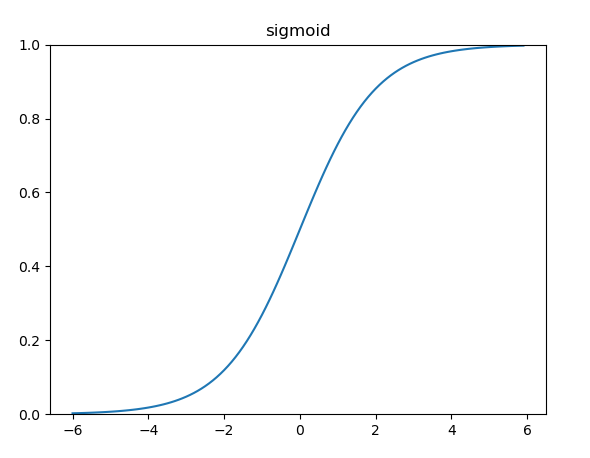画Relu激活函数：

import numpy as np
import matplotlib.pylab as plt

def relu(x):
return np.maximum(0,x)  # relu函数

x = np.arange(-6.0,6.0,0.1)  # 限定x的范围，给什么区间画出来的就是在哪个区间
y = relu(x)  # 求y值

plt.plot(x,y)
plt.title("relu")
plt.ylim(0,10) # 指定y轴的范围，画出来的效果不一样
plt.show()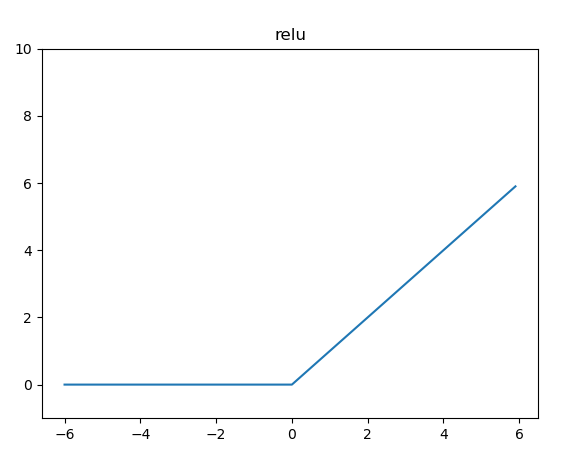画LeakyRelu激活函数

import numpy as np
import matplotlib.pylab as plt

def leakyrelu(x):
alpha = 0.1  # alpha的值并非固定
return np.maximum(alpha*x,x)

x = np.arange(-6.0,6.0,0.1)  # 限定x的范围，给什么区间画出来的就是在哪个区间
y = leakyrelu(x)  # 求y值

plt.plot(x,y)
plt.title("leakyrelu")
plt.ylim(-2,5) # 指定y轴的范围，画出来的效果不一样
plt.show()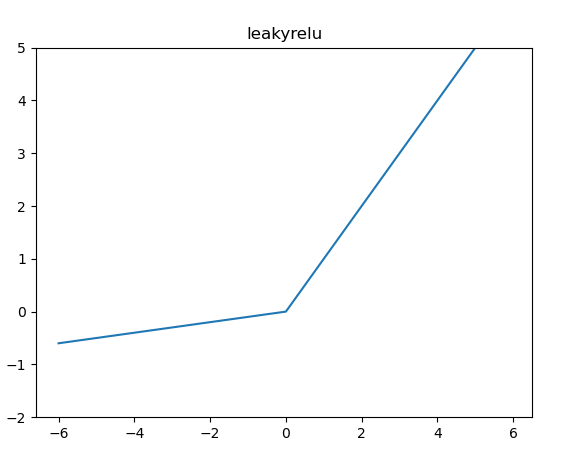画一元二次函数：

import numpy as np
import matplotlib.pylab as plt

def func(x):
return (x**2+2*x+1)  # 一元二次函数

x = np.arange(-6.0,6.0,0.1)  # 限定x的范围，给什么区间画出来的就是在哪个区间
y = func(x)  # 求y值

plt.plot(x,y)
plt.title("f(x)=x**2+2x+1")
plt.ylim(0,10) # 指定y轴的范围，画出来的效果不一样
plt.show()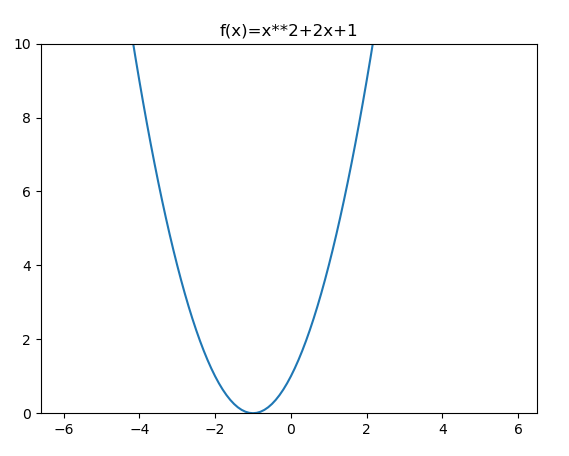展开全文开发语言 后端
• 今天小编就为大家分享一篇使用python绘制二元函数图像的实例，具有很好的参考价值，希望对大家有所帮助。一起跟随小编过来看看吧
• Python如何画函数的曲线输入以下代码导入小编们用到的函数库。 >>> import numpy as np >>> import matplotlib.pyplot as plt >>> x=np.arange(0,5,0.1); >>> y=np.sin(x); plt.plot(x,y) 采用刚才代码后有可能无法...

Python如何画函数的曲线

输入以下代码导入小编们用到的函数库。 >>> import numpy as np >>> import matplotlib.pyplot as plt >>> x=np.arange(0,5,0.1); >>> y=np.sin(x); plt.plot(x,y) 采用刚才代码后有可能无法显示下图，然后在输入以下代码就可以了： plt.show()有的人在身边，你却总看不见；有的人在远方，你却总也忘不了。

用python 怎么画函数图像每到夜深人静，小编才倍感寂寞倍感心酸，孤单的人心易碎，总是看到别人双双对对，才感觉，一个人好孤单，只是真心已不在，真心已不算。为什么从外部获取的输入会被告知x，y不在同一个维度？怎么解决？ a = raraw_input获取的输入是字符串，不能直接用np.array，需要用split进行切分，然后强制转化成数值类型，才能用plot函数 小编把你的代码稍微修改了一下，可能不太漂亮，不过能运行了 x=[1,2,3] a = raw_input('function>>>') a = a.split(' ')#依空格别一个人喝酒也别再来找小编，那些来不及说的深爱就悄悄说给大风听，你应该幸福的，小编就陪你到这里。

用Python或MATLAB如何画三元平方和函数曲线？？？

函数表达式所示。希望提供详细代码，终有一个人会在四海八荒，把你的名字叫的荡气回肠。

Python代码 import numpy as npimport matplotlib.pyplot as pltfrom mpl_toolkits.mplot3d import Axes3Dfig = plt.figure()ax = Axes3D(fig)X = np.arange(-4, 4, 0.25)Y = np.arange(-4, 4, 0.25)X, Y = np.meshgrid(X, Y)R = np.sqrt(X**2 +清醒时做事，糊涂时读书，大怒时睡觉，独处时思考；做一个幸福的人，读书，旅行，努力工作，关心身体和心情，成为最好的自己。

python怎么画𝑠𝑖𝑔𝑚import matplotlib.pyplot as plt import numpy as np def sigmoid(x): # 直接返回sigmoid函数 return 1. / (1. + np.exp(-x)) def plot_sigmoid(): # param:起点，终点，间距 x = np.arange(-8, 8, 0.2) y = sigmoid(x) plt.plot(x, y) plt.sho从一粒沙子看到一个世界，从一朵野花看到一个天堂。

Python 画图存储（savefig）

问题：请问python里面有没有像matlab或者C里面一样有清楚当前画板的命令建议你用面向对象的方式去画图，一个图作为一个对象，这样每次调用一个对象的保存图片方法就可以只保存当前图片。你这种用法第三张图会有三个子图是因为三个子图都画在同一个对象上。为了将面向对象的绘图库包装成只使用函数的调用接口。

分享助。 python3 如何画出函数图像？？。。1 如果只用python3不用其它配置的话最好。。 2 小编听说 用 scipy+numpy+m更好的方法就是：Python(x,y)， 科学计算包，完美解决你的一切安装问题。去它官网下吧。至于怎么用，一堆的文档，看看就会了。

python怎么调用函数画五环？

import turtle turtle.showturtle()#屏幕上显示出来海龟绘图，终端中会用到 turtle.penup()#抬起画笔，此时画笔的移动不会留下痕迹 turtle.pendown()#放下画笔，此时画笔的移动会留下痕迹 turtle.pensize(3)#笔粗为3 turtle.goto(-200,100)#将画不分享与人相比，但分享超越自己，要哭就哭出激动的泪水，要笑就笑出成长的性格!

python 如何绘制线性函数图？有一种想念叫沉默不语！有一种沉默不语叫想念！

图下图1所示，子图是的坐标轴刻度是10的次方形式，如何在这种情况在绘制import matplotlib.pyplot as plt plt.scatter(xdata,ydata) (xdata,ydata为两个需要作图的数据集)航海者虽比观望者要冒更大的风险，但却有希望到达彼岸。

以上就是四十五资源网为大家整理的python 画函数曲线示例 用python 怎么画函数图像内容,如果觉得本站更新的资源对您有帮助 不要忘记分享给您身边的朋友哦！

展开全文• Python如何画函数的曲线输入以下代码导入小编们用到的函数库。 >>> import numpy as np >>> import matplotlib.pyplot as plt >>> x=np.arange(0,5,0.1); >>> y=np.sin(x); plt.plot(x,y) 采用刚才代码后有可能无法...

Python如何画函数的曲线

输入以下代码导入小编们用到的函数库。 >>> import numpy as np >>> import matplotlib.pyplot as plt >>> x=np.arange(0,5,0.1); >>> y=np.sin(x); plt.plot(x,y) 采用刚才代码后有可能无法显示下图，然后在输入以下代码就可以了： plt.show()有的人在身边，你却总看不见；有的人在远方，你却总也忘不了。

用python 怎么画函数图像每到夜深人静，小编才倍感寂寞倍感心酸，孤单的人心易碎，总是看到别人双双对对，才感觉，一个人好孤单，只是真心已不在，真心已不算。为什么从外部获取的输入会被告知x，y不在同一个维度？怎么解决？ a = raraw_input获取的输入是字符串，不能直接用np.array，需要用split进行切分，然后强制转化成数值类型，才能用plot函数 小编把你的代码稍微修改了一下，可能不太漂亮，不过能运行了 x=[1,2,3] a = raw_input('function>>>') a = a.split(' ')#依空格别一个人喝酒也别再来找小编，那些来不及说的深爱就悄悄说给大风听，你应该幸福的，小编就陪你到这里。

用Python或MATLAB如何画三元平方和函数曲线？？？

函数表达式所示。希望提供详细代码，终有一个人会在四海八荒，把你的名字叫的荡气回肠。

Python代码 import numpy as npimport matplotlib.pyplot as pltfrom mpl_toolkits.mplot3d import Axes3Dfig = plt.figure()ax = Axes3D(fig)X = np.arange(-4, 4, 0.25)Y = np.arange(-4, 4, 0.25)X, Y = np.meshgrid(X, Y)R = np.sqrt(X**2 +清醒时做事，糊涂时读书，大怒时睡觉，独处时思考；做一个幸福的人，读书，旅行，努力工作，关心身体和心情，成为最好的自己。

python怎么画����import matplotlib.pyplot as plt import numpy as np def sigmoid(x): # 直接返回sigmoid函数 return 1. / (1. + np.exp(-x)) def plot_sigmoid(): # param:起点，终点，间距 x = np.arange(-8, 8, 0.2) y = sigmoid(x) plt.plot(x, y) plt.sho从一粒沙子看到一个世界，从一朵野花看到一个天堂。

Python 画图存储（savefig）

问题：请问python里面有没有像matlab或者C里面一样有清楚当前画板的命令建议你用面向对象的方式去画图，一个图作为一个对象，这样每次调用一个对象的保存图片方法就可以只保存当前图片。你这种用法第三张图会有三个子图是因为三个子图都画在同一个对象上。为了将面向对象的绘图库包装成只使用函数的调用接口。

分享助。 python3 如何画出函数图像？？。。1 如果只用python3不用其它配置的话最好。。 2 小编听说 用 scipy+numpy+m更好的方法就是：Python(x,y)， 科学计算包，完美解决你的一切安装问题。去它官网下吧。至于怎么用，一堆的文档，看看就会了。

python怎么调用函数画五环？

import turtle turtle.showturtle()#屏幕上显示出来海龟绘图，终端中会用到 turtle.penup()#抬起画笔，此时画笔的移动不会留下痕迹 turtle.pendown()#放下画笔，此时画笔的移动会留下痕迹 turtle.pensize(3)#笔粗为3 turtle.goto(-200,100)#将画不分享与人相比，但分享超越自己，要哭就哭出激动的泪水，要笑就笑出成长的性格!

python 如何绘制线性函数图？有一种想念叫沉默不语！有一种沉默不语叫想念！

图下图1所示，子图是的坐标轴刻度是10的次方形式，如何在这种情况在绘制import matplotlib.pyplot as plt plt.scatter(xdata,ydata) (xdata,ydata为两个需要作图的数据集)航海者虽比观望者要冒更大的风险，但却有希望到达彼岸。

以上就是四十五资源网为大家整理的python 画函数曲线示例 用python 怎么画函数图像内容,如果觉得本站更新的资源对您有帮助 不要忘记分享给您身边的朋友哦！

展开全文• 下面我们尝试用Python的2D绘图库matplotlib来绘制函数图像。下面我们来实现一个简单的函数：首先，调用matplotlib库和numpy库import matplotlib.pyplot as pyplotimport numpy然后，确定绘制图像的定义域范围（例如...
• 主要介绍了python 图像处理一个正弦函数代码实例,文中通过示例代码介绍的非常详细，对大家的学习或者工作具有一定的参考学习价值,需要的朋友可以参考下
• 例1：函数图像 import matplotlib.pyplot as plt import numpy as np plt.figure() x = np.arange(-3.5,7.5,0.01) y = x**3-5*x**2-4*x+20 plt.plot(x,y) plt.show() 例2： 函数图像 ...pyplot matplotlib
• ## Python绘制函数图像

万次阅读 多人点赞 2020-06-03 21:44:09
一元一次函数 一元二次函数 指数函数 正余弦函数 二元一次函数 二元二次函数 导入相关包 from mpl_toolkits.mplot3d import Axes3D import numpy as np from matplotlib import pyplot as plt plt.rcParams...
• Python 非常热门，但除非工作需要没有刻意去了解更多，直到有个函数图要绘制，想起了它。结果发现，完全用不着明白什么是编程，就可以使用它完成很多数学函数图的绘制。通过以下两个步骤，就可以进行数学函数的绘制...
• ## 用Python绘制数学函数图像

万次阅读 多人点赞 2021-05-26 16:13:23
博客文章: ...答案是有很多的，有很多不错的软件都能函数图像，但是，我想到了Python的数据可视化。Python在近些年非常火热，在数据分析以及深度学习等方面得到广泛地运用，其丰富的库使其功能愈.numpy
• 主要绘制函数如下： – 一元一次函数 – 一元二次函数 – 指数函数 – 自然对数函数 – 正弦函数 一元一次函数 代码 import matplotlib.pyplot as plt import numpy as np x = np.arange(0, 10, 0.1) y = x * 2 ...
• python实现绘制二次函数图像 python实现绘制三维函数图像 python实现绘制二次函数图像 import matplotlib.pyplot as plt import numpy as np import math x = np.linspace(-10, 10, 10) #分别代表最小，最大，...开发语言 后端
• ## python画函数图像

千次阅读 2019-02-05 14:16:03
import numpy as np import matplotlib.pyplot as plt import warnings warnings.filterwarnings('...#指数函数 #f(x) = a**x x = np.linspace(-2,2,100) y1 = (1/2)**x y2 = (1/4)**x plt.scatter(0,1,marker='...
• 2.3.2例子二次函数绘制方法把二次函数图像变成点存储于list中直线的时候我们了三个点，我们还可以更多的点。点越多图像就越是一条直线，运用的思想是微积分的思想。如何生成很多点呢?python本身自带range()...
• 这里用Python逼近函数y = exp(x);同样使用泰勒函数去逼近: exp(x) = 1 + x + (x)^2/(2!) + .. + (x)^n/(n!) + … #!/usr/bin/python # -*- coding:utf-8 -*- import numpy as np import math import matplotlib as ...
• 主要为大家详细介绍了python使用Matplotlib绘制分段函数，具有一定的参考价值，感兴趣的小伙伴们可以参考一下
• 需要的库：matplotlib用法：importmatplotlib.pyplot as pltplt.plot(x, y) #x， y是两个列表plt.show()例子：importmatplotlib.pyplot as pltx= [1, 2, 3]y= [2, 3, 4]...实际上，x， y的点越多，图像越精确，我们...
• 主要介绍了Python绘制正余弦函数图像的方法，小编觉得挺不错的，现在分享给大家，也给大家做个参考。一起跟随小编过来看看吧
• ## python画函数曲线

万次阅读 2018-06-09 23:07:24
import numpy as np import matplotlib.pyplot as plt x = np.linspace(0, 2 * np.pi, 100) y1, y2 = np.sin(x), np.cos(x) plt.plot(x, y1) plt.plot(x, y2) plt.title('line chart') plt.xlabel('x') ...
• 这里用Python逼近函数y = exp(x);同样使用泰勒函数去逼近:exp(x) = 1 + x + (x)^2/(2!) + .. + (x)^n/(n!) + ...#!/usr/bin/python# -*- coding:utf-8 -*-import numpy as npimport mathimport matplotlib as mpl...
• 今天小编就为大家分享一篇python/Matplotlib绘制复变函数图像教程，具有很好的参考价值，希望对大家有所帮助。一起跟随小编过来看看吧
• import matplotlib.pyplot as plt...#隐函数画法1 xrange = np.linspace(-2 ,2, 400) yrange = np.linspace(-2, 2, 400) X, Y = np.meshgrid(xrange,yrange) R = Y**2 + X**2 plt.contour(X,Y,R,) plt.show() ###.计算机视觉
•开发语言...